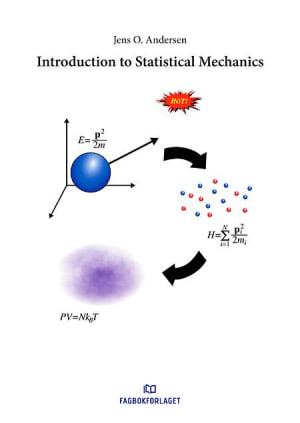Forfattere:
ISBN:
9788232101054
Utgitt:
Utgave:
1
Sider:
236
Introduction to Statistical Mechanics

## Introduction to Statistical Mechanics

"Introduction to Statistical Mechanics" is written for third-year university students in physics and engineering at The Norwegian University of Science and Technology. It is an introductory text to statistical mechanics that carefully develops new concepts and ideas from basic assumptions and established results. The prerequisites are mathematics courses on analysis and linear algebra as well as physics courses on classical mechanics, quantum mechanics, and thermodynamics. The first two chapters cover basic probability theory, Markov processes, and classical mechanics. Chapters three through five cover ensemble theory with applications to e .g spin systems, lattice vibrations, and black-body radiation. Chapter six is devoted to quantum statistical mechanics and the derivation of the Bose-Einstein and Fermi-Dirac distribution functions. The theory is applied to understand the fascinating white dwarfs, semiconductors, and the quark-gluon plasma. The book is equipped with an appendix, which covers some useful matematical material. Each chapter contains a number of exercises that are helpful in understanding the concepts.
Format
Språk
På lager - sendes nå
Antall
429,-